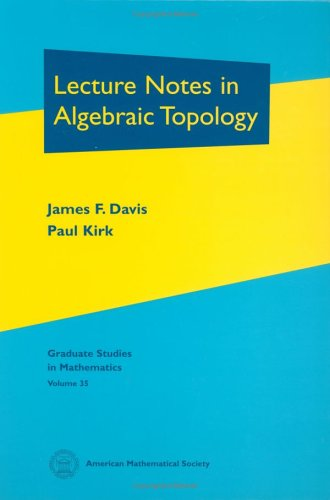Free Shipping in Australia# Lecture Notes in Algebraic Topology by Paul Kirk

In Stock
\$137.99
inc. GST
The amount of algebraic topology a graduate student specializing in topology must learn can be intimidating. This title bridges the gap between algebraic and geometric topology, both by providing the algebraic tools that a geometric topologist needs and by concentrating on those areas of algebraic topology that are geometrically motivated.
Only 1 left

## Lecture Notes in Algebraic Topology Summary

### Lecture Notes in Algebraic Topology by Paul Kirk

The amount of algebraic topology a graduate student specializing in topology must learn can be intimidating. Moreover, by their second year of graduate studies, students must make the transition from understanding simple proofs line-by-line to understanding the overall structure of proofs of difficult theorems. To help students make this transition, the material in this book is presented in an increasingly sophisticated manner. It is intended to bridge the gap between algebraic and geometric topology, both by providing the algebraic tools that a geometric topologist needs and by concentrating on those areas of algebraic topology that are geometrically motivated.Prerequisites for using this book include basic set-theoretic topology, the definition of CW-complexes, some knowledge of the fundamental group/covering space theory, and the construction of singular homology. Most of this material is briefly reviewed at the beginning of the book. The topics discussed by the authors include typical material for first- and second-year graduate courses. The core of the exposition consists of chapters on homotopy groups and on spectral sequences. There is also material that would interest students of geometric topology (homology with local coefficients and obstruction theory) and algebraic topology (spectra and generalized homology), as well as preparation for more advanced topics such as algebraic \$K\$-theory and the s-cobordism theorem.A unique feature of the book is the inclusion, at the end of each chapter, of several projects that require students to present proofs of substantial theorems and to write notes accompanying their explanations. Working on these projects allows students to grapple with the 'big picture', teaches them how to give mathematical lectures, and prepares them for participating in research seminars. The book is designed as a textbook for graduate students studying algebraic and geometric topology and homotopy theory. It will also be useful for students from other fields such as differential geometry, algebraic geometry, and homological algebra. The exposition in the text is clear; special cases are presented over complex general statements.

### Why buy from World of BooksOur excellent value books literally don't cost the earthFree delivery in AustraliaEvery used book bought is one saved from landfill

Chain complexes, homology, and cohomology Homological algebra Products Fiber bundles Homology with local coefficients Fibrations, cofibrations and homotopy groups Obstruction theory and Eilenberg-MacLane spaces Bordism, spectra, and generalized homology Spectral sequences Further applications of spectral sequences Simple-homotopy theory Bibliography Index.

GOR010312943
Lecture Notes in Algebraic Topology by Paul Kirk
Paul Kirk